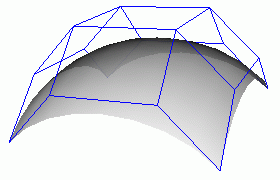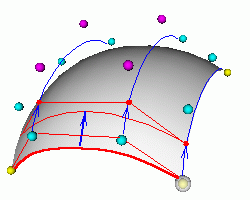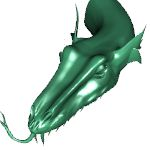# Tensor product spline surfaces

All spline curves studied before work well in space of any dimension. One need just take its control points in corresponding space. We used 2D plane as since it is enough and the simplest for computer representation.

## Bezier surface

Bezier spline surface (or patch) S(u,v) is constructed as the tensor product of two Bezier curves
S(u,v) = ∑i=0,nj=0,m Bin(u) Bjm(v)Pij ,     0 ≤ u, v ≤ 1 .
where (n+1)(n+1) control points Pij make the control polyhedron of the surface (see the left picture below).You can think that the tensor product patch is made by moving the control points of one Bezier curve (the red curve in the right picture) along a set of Bezier curves (the blue ones) to sweep out a surface
S(u,v) = ∑i=0,n Bin(u) ∑j=0,m Bjm(v)Pij = ∑i=0,n Bin(u) Pi (v),     Pi (v) = ∑j=0,m Bjm(v)Pij .
In every point of a Bezier patch we have two tangents   A = ∂S(u,v)/∂u and   B = ∂S(u,v)/∂v, therefore we get the normal to the surface N = [A B].
 N   N < 250 Move spline CPoints
Cubic Bezier spline patch   Use your fingers or mouse to control the model or Control Points (hold shift key or use mouse wheel to zoom it). Canvas is matched to your browser window. See also painted cubic Bezier patch.## 3D spline based models

The same cubic Bezier spline patches are used in 3D spline based models. Patches are generated by the old spline-based modelling tool "sPatch". I have found these models in Internet about 10 years ago.

Contents   Previous: NURBS   Next: Cubic Bezier patch with adaptive subdivision
updated 20 Mar 2013